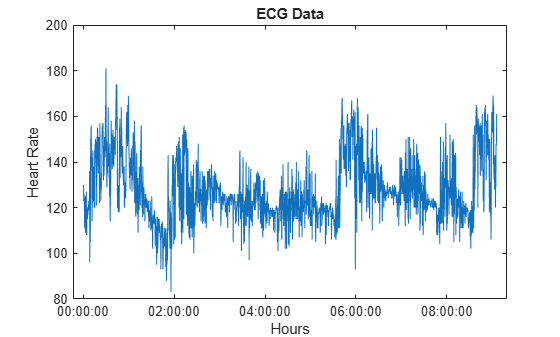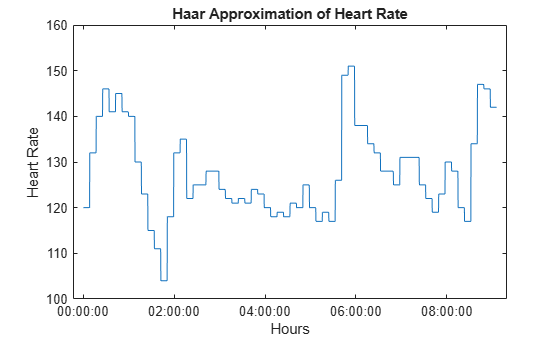haart

Haar 1-D wavelet transform

Description

example

[a,d] = haart(x) performs the 1-D Haar discrete wavelet transform of the even-length vector, x. The input x can be univariate or multivariate data. If x is a matrix, haart operates on each column of x. If the length of x is a power of 2, the Haar transform is obtained down to level log2(length(x)). Otherwise, the Haar transform is obtained down to level floor(log2(length(x)/2)).

example

[a,d] = haart(x,level) obtains the Haar transform down to the specified level.

example

[a,d] = haart(___,integerflag) specifies how the Haar transform handles integer-valued data, using any of the previous syntaxes.

Examples

collapse all

Obtain the Haar transform down to the default maximum level.

[a,d] = haart(wecg);

Obtain the Haar transform of a multivariate time series dataset of electricity consumption data down to level 4. The signals data is transposed so that each time series is in a column, rather than a row.

signals = signals';
[a,d] = haart(signals,4);

Obtain the Haar transform and inverse Haar transform of ECG heart rate data. The data is made up of integers only.

Load and plot the ECG data.

plot(times,HR)
xlabel('Hours')
ylabel('Heart Rate')
title('ECG Data')Obtain the Haar transform. Then, obtain the inverse Haar transform approximated at level 5. The scale for this level is 512 seconds, which is ${2}^{5}$ times the sampling interval (16 seconds).

[a,d] = haart(HR,'integer');
HaarHR = ihaart(a,d,5,'integer');

Compare the reconstructed data to the original data.

figure;
plot(times,HaarHR)
xlabel('Hours')
ylabel('Heart Rate')
title('Haar Approximation of Heart Rate')Input Arguments

collapse all

Input signal, specified as a vector or matrix. If x is a vector, it must be even length. If x is a matrix, each column must be even length, and haart operates on each column of x.

Data Types: single | double
Complex Number Support: Yes

Maximum level to which to perform the Haar transform, specified as a positive integer.

• If the length of x is a power of two, level is a positive integer less than or equal to log2(length(x)).

• If the length of x is even, but not a power of two, level is a positive integer less than or equal to floor(log2(length(x)/2)).

If level is 1, the detail coefficients, d, are returned as a vector or matrix, depending on whether the input is a vector or matrix, respectively.

Integer-valued data handling, specified as either 'noninteger' or 'integer'. 'noninteger' does not preserve integer-valued data in the Haar transform, and 'integer' preserves it. The 'integer' option applies only if all elements of the input, x, are integers. For integer-valued input, haart returns integer-valued wavelet coefficients. For both 'noninteger' and 'integer', however, the Haar transform algorithm uses floating-point arithmetic. If x is single precision, the Haar transform coefficients are single precision. For all other numeric type, the numeric type of the coefficients is double precision.

Output Arguments

collapse all

Approximation coefficients at the coarsest level, returned as a scalar, vector, or matrix of coefficients, depending on the level to which the transform is calculated. Approximation, or scaling, coefficients are a lowpass representation of the input. At each level, the approximation coefficients are divided into coarser approximation and detail coefficients.

Data Types: single | double

Detail coefficients, returned as a scalar, vector, matrix, or cell array. Detail coefficients are generally referred to as wavelet coefficients. The number of detail coefficients depends on the selected level and the length of the input. If d is a cell array, the elements of d are ordered from finest to coarsest resolution.

Note: Generated C and C++ code always returns the wavelet coefficients d in a cell array.

Data Types: single | double

Extended Capabilities

GPU ArraysAccelerate code by running on a graphics processing unit (GPU) using Parallel Computing Toolbox™.